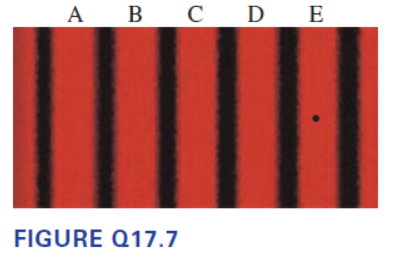# Problem: Figure Q17.7 shows the viewing screen in a double-slit experiment with monochromatic light.Fringe C is the central maximum. Suppose the wavelength of the light is 500 nm. How much farther is it from the dot on the screen in the center fringe E to the left slit than it is from the dot to the right slit?

###### FREE Expert Solution

Path difference:

$\overline{){\mathbf{∆}}{\mathbf{x}}{\mathbf{=}}{\mathbf{m}}{\mathbf{\lambda }}}$

Where m is the order and λ is the wavelength.

94% (367 ratings)###### Problem Details

Figure Q17.7 shows the viewing screen in a double-slit experiment with monochromatic light.

Fringe C is the central maximum.

Suppose the wavelength of the light is 500 nm. How much farther is it from the dot on the screen in the center fringe E to the left slit than it is from the dot to the right slit?